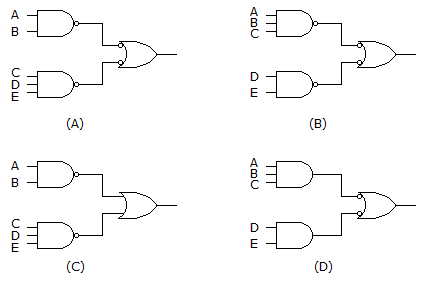# Digital Electronics - Combinational Logic Analysis - Discussion

### Discussion :: Combinational Logic Analysis - General Questions (Q.No.5)

5.

Implementing the expression AB + CDE using NAND logic, we get:[A]. (A) [B]. (B) [C]. (C) [D]. (D)

Answer: Option A

Explanation:

No answer description available for this question.

 Amit Kumar said: (Mar 3, 2015) Answer is C because only OR gate can perform + operation.

 Priya said: (May 21, 2015) Any one can explain me?

 Jai said: (Sep 26, 2015) But there is answer A? Is right or wrong?

 Md Nazim said: (Oct 5, 2015) Bubble at the end of and gate and bubble at the starting of or gate will cancel each other so A is correct answer. Because 1st and gate will give ab and 2nd and gate will give cde and or gate taking input 1st input=ab, 2nd input=cde will give the result ab+cde.

 Gaurav Chavda said: (Sep 4, 2016) A is the correct answer. Continuous 2 bubble cancels each other. So circuit in option A is simple to implement AB + CDE.

 Vineet said: (Jan 22, 2018) First of all, "read" the question. They asks using NAND gate. So option c having OR gate is canceled out, option D having AND gate also cancel out, now there are A and B. We are to find first AB we see option A has AB. So, accurately answer is A.

 Rohit said: (May 17, 2018) I am not understanding this. Please explain.

 Asharaf Ansari said: (Dec 7, 2018) No, we have to use only NAND gates.

 Paul said: (Jun 17, 2019) Yes, A is correct. (A+B)' + (CDE)' = from the output of NAN Gates. (A+B)+(CDE) = to the input of OR gate.

 Raji Oruganti said: (Jun 4, 2020) A is correct answer as per rule A"=A. (AB)"+(CDE)" =(AB)+(CDE).

 Kranti said: (Jul 6, 2020) The bubbled OR gate is equivalent to NAND gate, so(ab* (.)cde*)*= ab + cde.

#### Post your comments here:

Name *:

Email   : (optional)

» Your comments will be displayed only after manual approval.

#### Current Affairs 2021

Interview Questions and Answers